Next: Geometrical Tests Up: Eye Localization Previous: Eye Localization

## Detecting Eye Regions

The search space for the eye detection is now defined so we proceed to define the nature of the eye detection operation. In light of the highly symmetric, blob-like nature of the eye, we elect to use the symmetry transform to detect it as a peak in the interest map. Reisfeld proposes the use of a similar fixed size symmetry operator on the image . Similarly, we employ our more efficient symmetry transform (which also has a fixed size with its pre-specified annular sampling regions). Observe Tablefor the parameters of the annular regions for the symmetry transform at this stage. The usage of 8 different annular sampling regions with a wide range of radii is necessary due to the variety of sizes of the contours in the eye region. Large contours from the eye brows as well as small contours from the pupil are to be considered.

Table 3.2: The annular sampling regions to be used for eye detection
 Annular Region Number Range of Radii 1 0.75 < r < 2.25 pixels 2 1.75 < r < 3.25 pixels 3 2.75 < r < 4.25 pixels 4 3.75 < r < 5.25 pixels 5 4.75 < r < 7.25 pixels 6 6.75 < r < 9.25 pixels 7 8.75 < r < 11.25 pixels 8 10.75 < r < 14.25 pixels

Note that the symmetry transform has a fixed set of annular sampling regions. If the face is very large in the image and the eye region has a large pixel area, the annuli (which are at most 14 pixels in radius) will not overlap the whole eye region. However, since we know the approximate dimensions of the facial contour from the preceding stage, we know roughly what size the eye region must be. Figureshows a facial contour. The width of the facial contour is known (b) and thus, we expect the eye region to be at most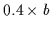in diameter. Ifis larger than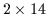pixels, then the eye region is larger than the symmetry operator (who has a maximum annular region radius of 14 pixels). Thus, the input image is scaled by a factor seyes so that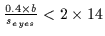. Unlike Reisfeld, we scale the image to accommodate the limited range of our fixed symmetry operator.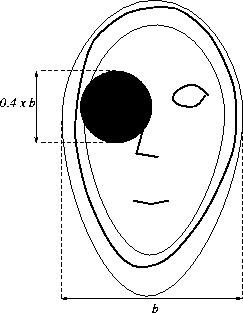Furthermore, the eye region, eyebrow, and eyelashes are surrounded by skin, and the iris is surrounded by the bright white sclera. Thus, we expect the eye region objects to be darker with respect to their immediate background and can restrict the computation of symmetry to dark symmetry only.

Figuredisplays the resulting peaks in the interest maps once the dark symmetry transform has been computed. Usually, the strongest peaks correspond to the eye regions. Thus we can limit the number of peaks to be processed further to the 5 strongest interest peaks3.1.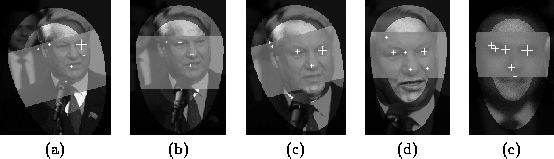The set of interest peaks (approximately 5) representing the possible eyes has been acquired. However, of these 5 peaks, which ones are the true eyes of the individual? It is possible to merely select the top two peaks in the eye band. Since eyes are such strong interest points, this is satisfactory in the majority of cases. However, it is sometimes possible that the top interest points are generated by other structures. For example, a loop of hair from the head could fall into the eye band and generate a strong interest peak (see Figure. Thus, we maintain the collection of possible eyes, accepting these false alarms for now. Further testing will isolate the true eyes from this set more reliably than the mere selection of the top two peaks.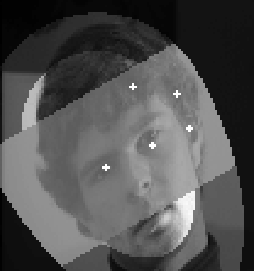We need to consider each pair of eyes in the set of detected peaks in the eye band. If 5 peaks are present, the total number of possible pairs is (25) = 10. However, we proceed by testing the strongest pairs first in a sequential manner until we find a pair that passes all tests. We can then stop testing any weaker pairs since we have converged to the two true eyes. Usually, the top two peaks will be the true eyes so we quickly converge without exhaustively testing all 10 pairs of possible eyes.Next: Geometrical Tests Up: Eye Localization Previous: Eye Localization
Tony Jebara
2000-06-23